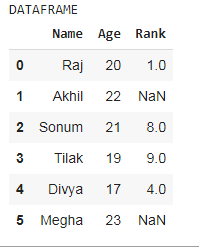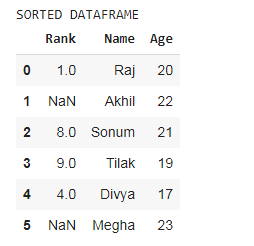Open in App
Not now

# How to sort a Pandas DataFrame by multiple columns in Python?

• Last Updated : 25 Aug, 2021

Sorting is one of the operations performed on the dataframe based on conditional requirements. We can sort dataframe alphabetically as well as in numerical order also. In this article, we will see how to sort Pandas Dataframe by multiple columns.

Method 1: Using sort_values() method

Syntax: df_name.sort_values(by column_name, axis=0, ascending=True, inplace=False, kind=’quicksort’, na_position=’last’, ignore_index=False, key=None)

Parameters:

by: name of list or column it should sort by

axis: Axis to be sorted.(0 or ‘axis’  1 or ‘column’) by default its 0.(column number)

ascending: Sorting ascending or descending. Specify lists of bool values for multiple sort orders. The list of bool values must match the no. of values of ‘by’ i.e. column_names. By default it is true.

inplace: By default it is false. but if its value is true it performs operation in-place i.e. in proper place.

kind: Choice of sorting algorithm like quick sort. merge sort, heap sort. by default it is quick sort.

Sorting a DataFrame:

• Import module.
• Create a DataFrame.
• Now, sort a DataFrame using the above syntax.

Creating a DataFrame:

## Python3

 `#import libraries``import` `numpy as np``import` `pandas as pd` `# creating a dataframe``df ``=` `pd.DataFrame({``'Name'``: [``'Raj'``, ``'Akhil'``, ``'Sonum'``, ``'Tilak'``, ``'Divya'``, ``'Megha'``],``                   ``'Age'``: [``20``, ``22``, ``21``, ``19``, ``17``, ``23``],``                   ``'Rank'``: [``1``, np.nan, ``8``, ``9``, ``4``, np.nan]})` `# printing the dataframe``print``(``'DATAFRAME'``)``df`

Output:Example 1:

## Python3

 `# using the sorting function``print``(``'SORTED DATAFRAME'``)``df.sort_values(by``=``[``'Age'``], ascending``=``False``)`

Output:In the above example the ascending value is false so, DataFrame is sorted into descending order.

Example 2:

## Python3

 `print``(``'SORTED DATAFRAME'``)``df.sort_values(by ``=` `[``'Rank'``, ``'Age'``], ascending ``=` `[``True``, ``False``], na_position ``=` `'first'``)`

Output:In the above example the DataFrame is sorted according to ‘Rank’ column and the nan values are positioned at the first.

Example 3:

## Python3

 `print``(``'SORTED DATAFRAME'``)``df.sort_values(by ``=` `[``'Name'``, ``'Rank'``], axis``=``0``, ascending``=``[``False``, ``True``], inplace``=``False``,``               ``kind``=``'quicksort'``, na_position``=``'first'``, ignore_index``=``True``, key``=``None``)`

Output:In the above example the dataframe is sorted based on the ‘Rank’ column, but the index number is started with 0 because we have given parameter ‘ignore_index = True’. In other examples the index is unordered because we have not given ‘ignore_index’ parameter.

Method 2: Using sort_index() method

Syntax:

df_name.sort_index(axis=0, level=None, ascending=True, inplace=False, kind=’quicksort’, na_position=’last’, sort_remaining=True, ignore_index=False, key=None)

Example 1: Using the above created dataframe

## Python3

 `print``(``'SORTED DATAFRAME'``)``df.sort_index(ascending``=``False``)`

Output:The index of the DataFrame is in descending order because the value of ascending parameter is False. The DataFrame is sorted in order of index.

Example 2:

## Python3

 `print``(``'SORTED DATAFRAME'``)``df.sort_index(axis``=``1``, ascending``=``False``)`

Output:My Personal Notes arrow_drop_up